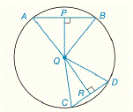Chapter 6.CR, Problem 35CRElementary Geometry For College St...

7th Edition
Alexander + 2 others
ISBN: 9781337614085

Solutions

Chapter
SectionElementary Geometry For College St...

7th Edition
Alexander + 2 others
ISBN: 9781337614085
Textbook Problem

In ⊙ Q with ∆ A B Q and ∆ C D Q , m ∠ A B ^ > m ∠ C D ^ . Also, Q P ^ A B ^ a n d   Q R ^ C D ^ .a) How are A B and C D related?b) How are Q P and Q R related?c) How are m ∠ A and m ∠ C related?To determine

a)

To Find: How are AB and CD related?

Explanation

Theorem:

1) In a circle (or in congruent circles) containing two unequal chords, the shorter chord is at the greater distance from the center of the circle..

2) In a circle (or in congruent circles) containing two unequal chords, the chord nearer the center of the circle has the greater length.

3) In a circle (or in congruent circles) containing two unequal chords, the longer chord corresponds to the greater minor arc.

4) In a circle (or in congruent circles) containing two unequal minor arcs, the greater minor arc corresponds to the longer chords related to these arcs.

Calculation:

Given that In Q with ABQ and CDQ, m&#

To determine

b)

To Find: How are QP and QR related?

To determine

c)

To Find: How are mA and mC related?

Still sussing out bartleby?

Check out a sample textbook solution.

See a sample solution

The Solution to Your Study Problems

Bartleby provides explanations to thousands of textbook problems written by our experts, many with advanced degrees!

Get Started

Find all solutions of the equation. 20. x4 = 1

Single Variable Calculus: Early Transcendentals, Volume I

37. Sketch a graph of each of the following basic functions. (a) (b) (c)

Mathematical Applications for the Management, Life, and Social Sciences

Change 4 ft to inches.

Elementary Technical Mathematics

Find the limit. lim0cos1sin

Single Variable Calculus: Early Transcendentals

True or False: converges absolutely.

Study Guide for Stewart's Multivariable Calculus, 8th

In the graph at the right; limx2f(x)=. a) 2 b) 4 c) 0 d) does not exist

Study Guide for Stewart's Single Variable Calculus: Early Transcendentals, 8th